Home > INT3 > Chapter 11 > Lesson 11.2.1 > Problem11-62

11-62.
1.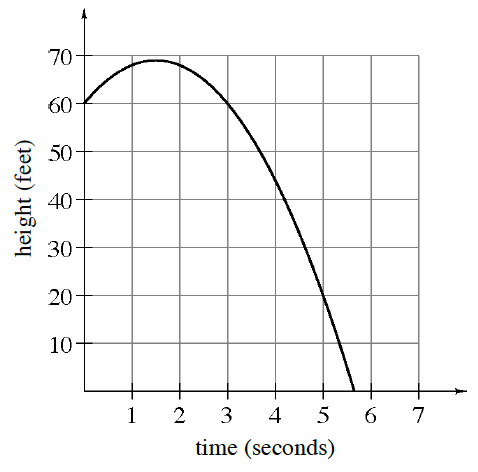The height h (in feet) of object A after t seconds is given by the equation h = 80t – 16t2. The height of object B is given by the graph at right. Homework Help ✎

1. Which object was in the air longer? How long was this object in the air?

2. Which object traveled higher? How high did it travel?

3. For what times is the height of object A higher than 64 feet?

4. In the context of this problem, what domain is reasonable for the function that describes object A?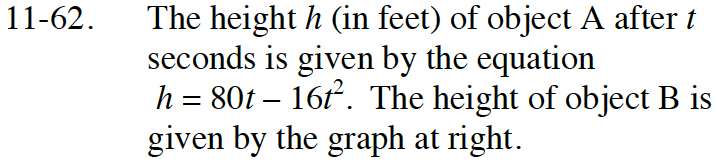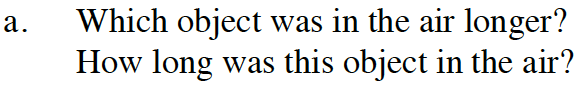Find the x-intercepts for object A to determine how long it was in the air.

Object A was in the air for 5 seconds.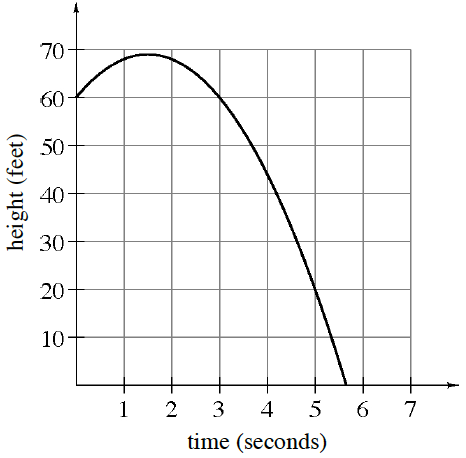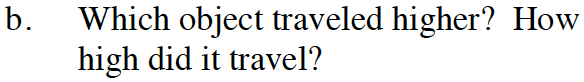Average the x-intercepts for object A to find the vertex.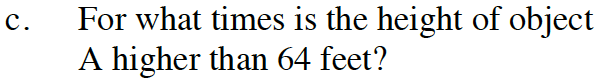Solve 80t − 16t2 > 64

1 < t < 4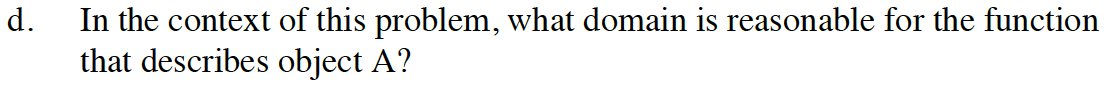For what values of t is the ball on or above the ground?